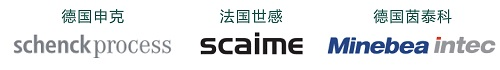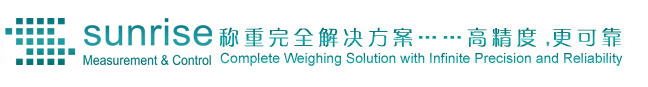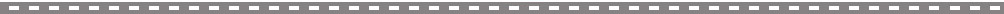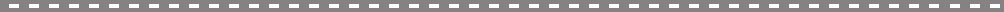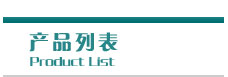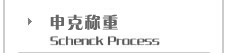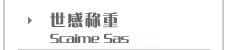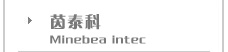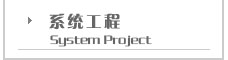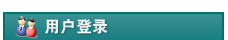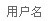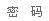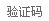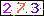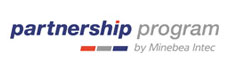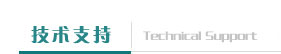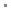网站首页 > 技术支持休闲文摘 更多…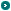有趣的计算

Hard work(努力工作)      H+A+R+D+W+O+R+K=8+1+18+4+23+15+18+11=98

Knowledge(知识)           K+N+O+W+L+E+D+G+E=11+14+15+23+12+5+4+7+5=96

Luck(运气)                    L+U+C+K=12+21+3+11=47

Attitude(态度)                A+T+T+I+T+U+D+E=1+20+20+9+20+21+4+5=100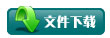电话：(021) 6806 0812   传真：(021) 5429 2128     邮件：info@ssmcc.com 版权所有  上海赛锐测控工程有限公司 @ 2004-2020      沪 ICP 备  12021431号